CUET  >  Algebra: Functions Questions - 1

# Algebra: Functions Questions - 1 - General Test Preparation for CUET

Question 1: How many onto functions can be defined from the set A = {1, 2, 3, 4} to {a, b, c}?

A. 81

B. 79

C. 36

D. 45

Explanation.

First let us think of the number of potential functions possible. Each element in A has three options in the co-domain. So, the number of possible functions = 34 = 81.
Now, within these, let us think about functions that are not onto. These can be under two scenarios.
Scenario 1: Elements in A being mapped on to exactly two of the elements in B (There will be one element in the co-domain without a pre-image).
Let us assume that elements are mapped into A and B. Number of ways in which this can be done = 24 – 2 = 14
24 because the number of options for each element is 2. Each can be mapped on to either A or B
-2 because these 24 selections would include the possibility that all elements are mapped on to A or all elements being mapped on to B. These two need to be deducted.
The elements could be mapped on B & C only or C & A only. So, total number of possible outcomes = 14 * 3 = 42.
Scenario 2: Elements in A being mapped to exactly one of the elements in B. (Two elements in B without pre-image). There are three possible functions under this scenario. All elements mapped to a, or all elements mapped to b or all elements mapped to c.
Total number of onto functions = Total number of functions – Number of functions where one element from the co-doamin remains without a pre-image - Number of functions where 2 elements from the co-doamin remain without a pre-image.
⇒ Total number of onto functions = 81 – 42 – 3 = 81 – 45 = 36

The question is "How many onto functions can be defined from the set A = {1, 2, 3, 4} to {a, b, c}?"

##### Hence the answer is "36"

Choice C is the correct answer.

Question 2Find the maximum value of f(x); if f(x) is defined as the Min {-(x – 1)2 + 2, (x – 2)2 + 1}

A. 1

B. 2

C. 0

D. 3

Explanation.

First let us find the range where Min (-(x – 1)2 + 2, (x – 2)2 + 1) is – (x – 1)2 + 2.
In other words, in which range is – (x – 1)2 + 2 < (x – 2)2 + 1.
–(x2 – 2x +1) + 2 < x2 – 4x + 4 + 1
0 < 2x2 – 6x + 4
x2 – 3x + 2 > 0
(x – 1) (x – 2) > 0
⇒ x > 2 or x < 1
So, for x ∈ (1, 2) , f(x) = (x – 2)2 + 1
And f(x) = –(x – 1)2 + 2 elsewhere.
Let us also compute f(1) and f(2)
f(1) = 2, f(2) = 1
For x ∈ (-∞, 1), f(x) = –(x – 1)2 + 2
f(1) = 2
For x ∈ (1, 2), f(x) = (x – 2)2 + 1
f(2) = 1
For x ∈ (2, ∞), f(x) = –(x – 1)2 + 2
For x < 1 and x > 2, f(x) is -(square) + 2 and so is less than 2.
When x lies between 1 and 2, the maximum value it can take is 2. f(1) = 2 is the highest value f(x) can take.
As a simple rule of thumb, the best way to approach this question is to solve the two expressions. This gives us the meeting points of the two curves. One of the two meeting points should be the maximum value.

The question is "Find the maximum value of f(x); if f(x) is defined as the Min {-(x – 1)2 + 2, (x – 2)2 + 1}?"

##### Hence the answer is "2"

Choice B is the correct answer.

Question 3: Consider functions f(x) = x2 + 2x, g(x) = √(x + 1) and h(x) = g(f(x)). What are the domain and range of h(x)?

Answer. Domain: (-∞, +∞), Range -[0, ∞]

Explanation.

h(x) = g(f(x)) = g(x2 + 2x) = √(x2 + 2x + 1) = √(x + 1)2 = |x + 1|
This bit is very important, and often overlooked.
√(9) = 3, not ±3
If x2 = 9, then x can be ±3, but √(9) is only +3.
So, √(x2) = |x|, not +x, not ±x
Domain of |x + 1| = (-∞, +∞), x can take any value.
As far as the range is concerned, |x + 1| cannot be negative. So, range = [0, ∞)

The question is "What are the domain and range of h(x)?"

##### Hence the answer is "Domain: (-∞, +∞), Range -[0, ∞]"

Question 4: [x] = greatest integer less than or equal to x. If x lies between 3 and 5, 5 inclusive, what is the probability that [x2] = [x]2?

A. Roughly 0.64

B. Roughly 0.5

C. Roughly 0.14

D. Roughly 0.36

Explanation. Let us take a few examples.
 = 2
[3.52] = 12 [3.5]2 = 9
 = 16 2 = 16
For x ∈ (3, 5). [x]2 can only take value 9, 16 and 25.
Let us see when [x2] will be 9, 16 or 25.
If [x2] = 9,
x2 ∈ [9, 10)
x ∈ [3, √10)
[x2] = 16
x2 ∈ [16, 17)
x ∈ [4, √17)
In the given range [x2] = 25 only when x = 5
So [x2] = [x]2 when x ∈ [3, √10] or [4, √10) or 5.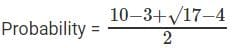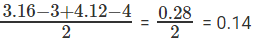The question is "If x lies between 3 and 5, 5 inclusive, what is the probability that [x2] = [x]2?"

##### Hence the answer is "Roughly 0.14"

Choice C is the correct answer.

Question 5: Give the domain and range of the following functions:

A. f(x) = x2 + 1

B. g(x) = log(x + 1)

C. h(x) = 2x

D. f(x) = 1/x+1

E. p(x) = |x + 1|

F. q(x) = [2x], where [x] gives the greatest integer less than or equal to x

Explanation.

A. f(x) = x2 + 1
Domain = All real numbers (x can take any value)
Range [1, ∞). Minimum value of x2 is 0.
B. g(x) = log (x + 1)
Domain = Log of a negative number is not defined so (x + 1) > 0 or x > -1
Domain (-1, ∞)
Range = (-∞, +∞)
Note: Log is one of those beautiful functions that is defined from a restricted domain to all real numbers. Log 0 is also not defined. Log is defined only for positive numbers
C. h(x) = 2x Domain - All real numbers.
Range = (0, ∞)
The exponent function is the mirror image of the log function.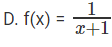Domain = All real numbers except -1
Range = All real numbers except 0.
E. p(x) = |x + 1|
Domain = All real numbers
Range = [0, ∞) Modulus cannot be negative
F. q(x) = [2x], where [x] gives the greatest integer less than or equal to x
Domain = All real numbers
Range = All integers
The range is NOT the set of even numbers. [2x] can be odd. [2 * 0.6] = 1. It is very important to think fractions when you are substituting values.

Question 6: How many elements are present in the domain of 9–xCx+1?

A. 5

B. 6

C. 4

D. 7

Explanation.

For nCr to be defined, we should have r greater than or equal to zero and, n greater than or equal to r.
Therefore 9 – x ≥ x + 1 or 4 ≥ x and
also x + 1 > 0 or x > –1
Therefore x takes on the values {–1,0,1,2,3,4}
Therefore domain = {–1,0,1,2,3,4} and range = {10C0,9C1,8C2,7C3,6C4,5C5}
There are 6 elements in the domain.

The question is "How many elements are present in the domain of 9–xCx+1? "

##### Hence the answer is "6"

Choice B is the correct answer.

Question 7: f(x + y) = f(x)f(y) for all x, y, f(4) = + 3 what is f(–8)?

A. 1/3

B. 1/9

C. 9

D. 6

Explanation.

f(x + 0) = f(x) f(0)
f(0) = 1
f(4 + – 4) = f(0)
f(4 + – 4) = f(4) f(–4)
1 = +3 * f(–4)

f(-4) =  1/3

f(– 8) = f(– 4 + (– 4)) = f(– 4) f(– 4)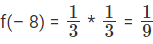The question is "f(x + y) = f(x)f(y) for all x, y, f(4) = + 3 what is f(–8)?"

##### Question 8: If f(x – 3) = 2x3 + p – qx and f(x2 – 4) = x2 – 8q + 6p, then what is the value of p – q?

A. 5

B. 10

C. 6

D. Cannot determine

Explanation.

f(x – 3) = 2x3 + p – qx
Let x = 3, then f(0) = 54 + p – 3q ---- (1)
f(x2 – 4) = x2 – 8q + 6p
Let x2 = 4, then f(0) = 4 – 8q + 6p --- (2)
From (1) and (2)
54 + p – 3q = 4 – 8q + 6p
50 = 5p – 5q
p - q = 10

The question is "If f(x – 3) = 2x+ p – qx and f(x– 4) = x– 8q + 6p, then what is the value of p – q?"

##### Hence the answer is "10"

Choice B is the correct answer.

Question 9: Given that x is real and f(x) = f(x + 1) + f(x – 1). Determine the value of ‘a’ that will satisfy f(x) + f(x + a) = 0

A. -1

B. -2

C. 1

D. 3

Explanation.

f(x) = f(x + 1) + f(x – 1)
Let f(x) = p and f(x – 1) = q
f(x + 1) = f(x + 1 + 1) + f(x + 1 – 1) (Put x = x + 1 here!)
= f(x + 2) + f(x)
p – q = f(x + 2) + p
Or f(x + 2) = -q
f(x + 2) = f(x + 2 + 1) + f(x + 2 – 1) (Put x = x + 2 here!)
= f(x + 3) + f(x + 1)
– q = f(x + 3) + p – q
Or f(x + 3) = -p
At this point we notice that f(x) = p and f(x+3) = -p
f(x) + f(x + 3) = 0 (This is the condition to be satisfied to determine a)
Hence ‘a’ = 3

The question is "Given that x is real and f(x) = f(x + 1) + f(x – 1). Determine the value of ‘a’ that will satisfy f(x) + f(x + a) = 0?"

##### Hence the answer is "3"

Choice D is the correct answer.

Question 10x is a real number such that f(x) = 1/x when x > 0 and f(x) = 1/x+1 otherwise. Also fn(x) = f(fn - 1 (x)). What is f(3) + f2(-3) + f3(3) + f4(-3)?

A. -2/3

B. 14/3

C. 0

D. 3

Explanation.

f(x) = 1/x = when x > 0; f(x) = 1/x+1 otherwise

f(3) = 1/3

f2(3) = f(f(3) = f (1/3) = 3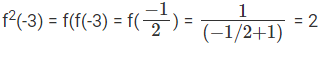f(3) + f2(-3) + f3(3) + f4(-3)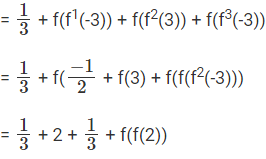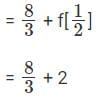= 14/3

The question is "What is f(3) + f2(-3) + f3(3) + f4(-3)?"

##### Hence the answer is "14/3"

Choice B is the correct answer.

Question 11: Which of the following functions are identical?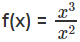g(x) = (√x)2
h(x) = x

A. f(x) and g(x)

B. f(x) and h(x)

C. All 3 are identical

D. None of these

Explanation.

For functions to be identical, their domains should be equal
f(x) – x can’t be zero
g(x) – x can’t be negative
h(x) – x can take all possible values

The question is "Which of the given functions are identical?"

##### Hence the answer is "None of these are identical"

Choice D is the correct answer.

The document Algebra: Functions Questions - 1 | General Test Preparation for CUET is a part of the CUET Course General Test Preparation for CUET.
All you need of CUET at this link: CUET

## General Test Preparation for CUET

176 videos|328 docs|399 tests

## FAQs on Algebra: Functions Questions - 1 - General Test Preparation for CUET

 1. What is a function in algebra?Ans. A function in algebra is a relation between a set of inputs, called the domain, and a set of outputs, called the range, such that each input has exactly one corresponding output. It can be represented as f(x), where x is the input variable and f(x) is the corresponding output.
 2. How do you determine if a relation is a function?Ans. To determine if a relation is a function, you need to check if each input in the domain has a unique output in the range. One way to do this is by using the vertical line test. If a vertical line intersects the graph of the relation at more than one point, then it is not a function. Otherwise, if each vertical line intersects the graph at most one point, then the relation is a function.
 3. How do you find the domain and range of a function?Ans. To find the domain of a function, you need to identify all the possible values for the input variable that make the function defined. The range, on the other hand, is the set of all possible output values that the function can produce. The domain and range can be determined by analyzing the graph of the function or by looking at the restrictions on the input variable and the possible output values.
 4. What is the difference between a linear function and a quadratic function?Ans. A linear function is a function that can be represented by a straight line graph, where the output values increase or decrease at a constant rate. On the other hand, a quadratic function is a function that can be represented by a parabolic graph, where the output values increase or decrease at a non-constant rate. In a quadratic function, the highest power of the input variable is 2, whereas in a linear function, the highest power is 1.
 5. How do you solve equations involving functions?Ans. To solve equations involving functions, you need to isolate the output variable on one side of the equation. This can be done by applying inverse operations, such as addition, subtraction, multiplication, or division, to both sides of the equation. Once the output variable is isolated, you can substitute known input values to find the corresponding output values.

## General Test Preparation for CUET

176 videos|328 docs|399 testsExplore Courses for CUET examSignup to see your scores go up within 7 days! Learn & Practice with 1000+ FREE Notes, Videos & Tests.
10M+ students study on EduRev
Track your progress, build streaks, highlight & save important lessons and more!
Related Searches

,

,

,

,

,

,

,

,

,

,

,

,

,

,

,

,

,

,

,

,

,

;